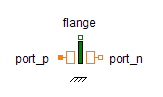Real To Integer - MapleSim Help

Real To Integer

Convert Real to Integer signalDescription The Real To Integer component converts the real input to the nearest integer value.Equations $y=\left\{\begin{array}{cc}⌊u+\frac{1}{2}⌋& u>0\\ ⌈u-\frac{1}{2}⌉& \mathrm{otherwise}\end{array}$Connections

 Name Description Modelica ID $u$ Real input signal u $y$ Integer output signal yModelica Standard Library The component described in this topic is from the Modelica Standard Library. To view the original documentation, which includes author and copyright information, click here.